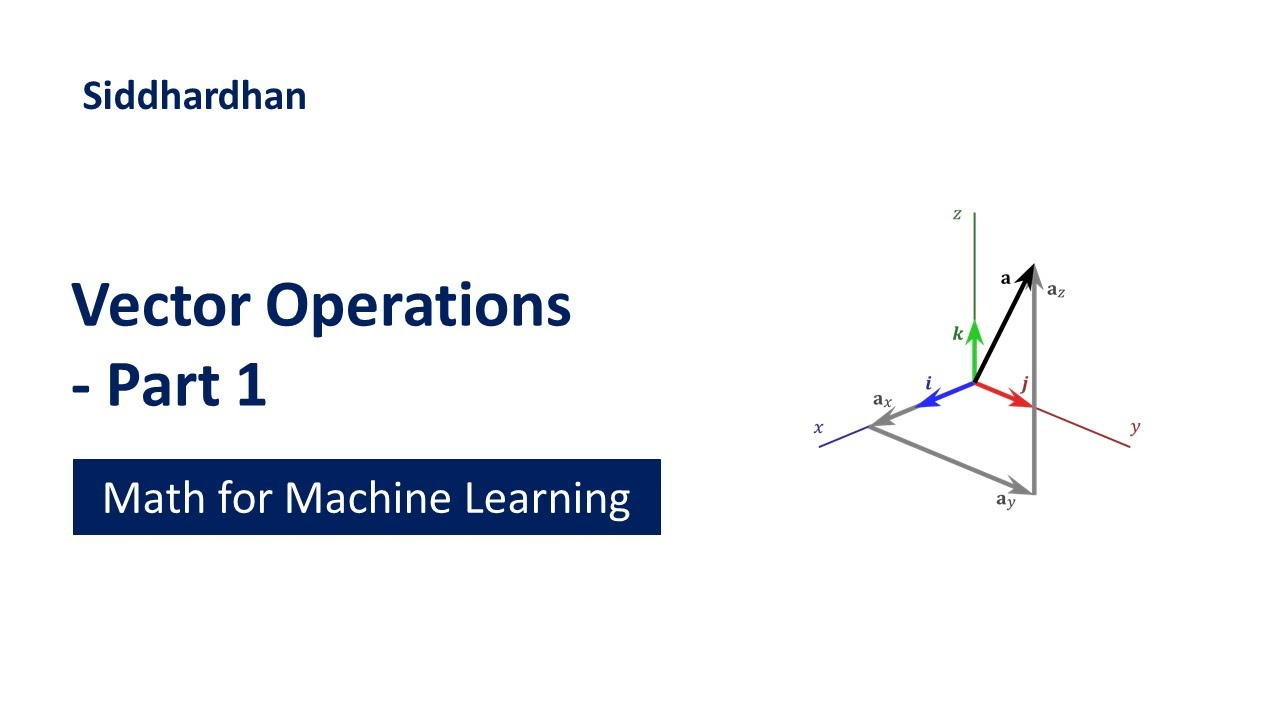1620372000

# Vector Operations - Part 1 | Mathematics for Machine Learning | Linear Algebra

This video is about vector operations such as vector addition, vector subtraction, multiplying a vector by a scalar and Angle between two Vectors. Vector Operations is one of the important topics in Linear Algebra for Machine Learning.